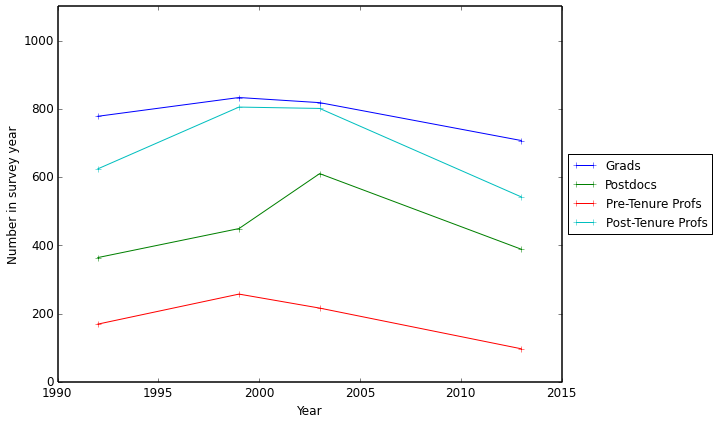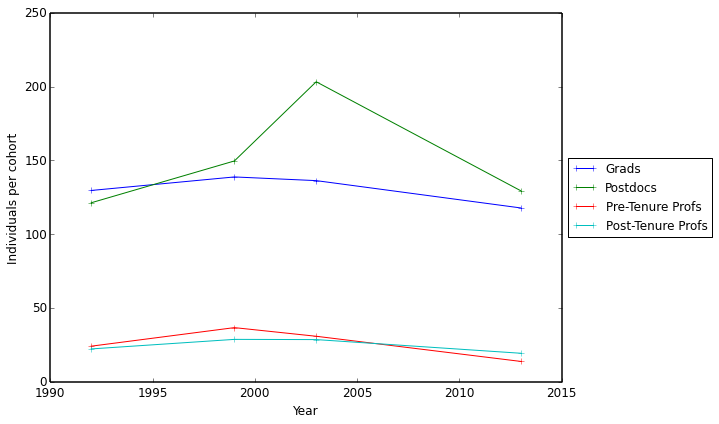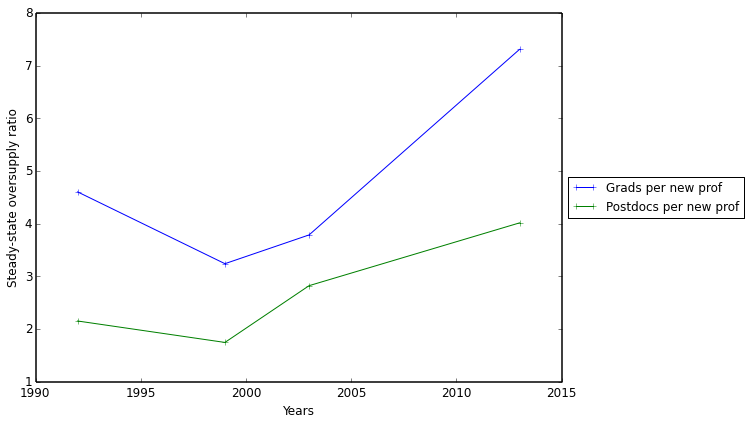### How is the US astronomy career pipeline changing?

Recently, the American Astronomical Society’s Committee on the Status of Women in Astronomy (CSWA) released a report on the demographics of US astronomers throughout the academic career cycle: graduate students, postdocs, and the various ranks of professors.  The major goal of the report (written by my friend, Prof. Meredith Hughes) was to assess the progress of women through the “pipeline” as a function of time: are women moving into the professor level in proportion to their increasing representation at the graduate student and postdoc levels, or are they “leaking out” of the pipeline?  The full report, summarized in this blog post, addresses this important question.

I was interested in a more basic question: how has the size of the pipeline itself changed over time?  That is, how many more (or fewer) grad students and postdocs are working in US astronomy compared to the number of professors over time?  The report provides proportions of the total number of men and women at each career stage by year (in 1992, 1999, 2003, and 2013), but I was curious about the totals.  Since the survey only covers a limited sample of institutions, it doesn’t represent the totality of the US astronomy job market, but it should provide a useful look.

In :
```%matplotlib inline
import pandas
import matplotlib.pyplot as plt
import matplotlib.pylab as pylab

pylab.rcParams['figure.figsize'] = (8, 6)```

Below is the data from Figures 2 and 3 of the CWSA report. Because the 2013 report adds 8 universities and 3 research institutes to the 32 institutions surveyed in previous years, I scale the 2013 values down by that fraction. Without the raw data, it’s hard to know whether adding the additional institutions (such as Goddard) bias the career stage proportion relative to previous years. The direct scaling below should provide at least a basic level of year-to-year consistency.

In :
```scale_2013 = 32./(32+8+3)
"postdoc":[63,90,137,145],"assistant":[29,45,34,34],
"associate":[18,37,40,44],"full":[23,37,60,71]})
"postdoc":[301,359,473,377],"assistant":[140,212,182,96],
"associate":[162,220,157,187],"full":[421,511,544,426]})
df = df_men + df_women
df.index = df['year']/2
del(df['year'])
df.ix[df.index==2013] *= scale_2013
df```
Out:
year
1992 169.000000 180.000000 444.000000 778.000000 364.000000
1999 257.000000 257.000000 548.000000 833.000000 449.000000
2003 216.000000 197.000000 604.000000 818.000000 610.000000
2013 96.744186 171.906977 369.860465 706.976744 388.465116

4 rows × 5 columns

In :
```def outside_legend():
# Shink current axis by 20%
ax=plt.gca()
box = ax.get_position()
ax.set_position([box.x0, box.y0, box.width * 0.8, box.height])

# Put a legend to the right of the current axis
ax.legend(loc='center left', bbox_to_anchor=(1, 0.5))```

First, I plot total numbers of astronomers (men + women) in each career stage by survey year. I’ve lumped the “associate” and “full” professor categories together as “post-tenure,” although this may not be appropriate at all institutions. Note also that for research institutions, “professor” may indicate a staff position.

In :
```plt.plot(df.index,df["grad"],"+-",label="Grads")
plt.plot(df.index,df["postdoc"],"+-",label="Postdocs")
plt.plot(df.index,df["assistant"],"+-",label="Pre-Tenure Profs")
plt.plot(df.index,(df["associate"]+df["full"]),"+-",label="Post-Tenure Profs")
plt.ylim(0,1100)
plt.xlabel("Year")
plt.ylabel("Number in survey year")
outside_legend()```To my surprise, the number of astronomers of all career stages appears to have declined relative to a peak in the early 2000s, presumably reflecting flat funding profiles in the US and the lingering effects of the financial crisis.

Comparing raw numbers is somewhat misleading, however, as professors remain in that career stage far longer than postdocs and grad students. We can get a sense of the size of a “cohort” by dividing by a rough average number of years that astronomers remain in a career stage before moving on (or out of the academic pipeline). I have used 6 years for grad students, 3 for postdocs (although taking a second postdoc has become increasingly common in the last two decades), 7 for pre-tenure professors, and 28 for tenured professors.  Dividing by these values gives the rough number of individuals entering (and leaving, in steady state) a given career stage each year.

In :
```plt.plot(df.index,df["grad"]/6.,"+-",label="Grads")
plt.plot(df.index,df["postdoc"]/3.,"+-",label="Postdocs")
plt.plot(df.index,df["assistant"]/7.,"+-",label="Pre-Tenure Profs")
plt.plot(df.index,(df["associate"]+df["full"])/28.,"+-",label="Post-Tenure Profs")
plt.xlabel("Year")
plt.ylabel("Individuals per cohort")
outside_legend()```This plot highlights a large excess of postdocs in the early 2000s, presumably due to the influx of funds from to NASA’s Great Observatories (Chandra launched in 1999 and Spitzer in 2003).

Finally, we come to the sticky question: is the pipeline to professorship wider or narrower than it used to be?

Ideally, one would track a defined group through time–surveying the career outcomes of an unbiased sample of PhDs every five years, for example. The CSWA report performs survival analysis using the 1992/2003 and 2003/2013 survey pairs, but the format of the survey can’t ensure that those counted as grads or postdocs at one of the survey periods are among those being counted in the next. (A precocious senior grad student in 2003 could well be tenured by 2013, but would not be counted as “surviving” into the assistant professor stage.)

A more direct means of assessing the width of the pipeline is to compare the relative proportions of grads, postdocs, and assistant professors at each survey interval and assume a steady state. That is, if we keep graduating PhDs, hiring postdocs, and hiring assistant professors/research staff at the rate we are today, what is the oversupply ratio? This is the value most of interest to students already in the pipeline, as it indicates the amount of competition for permanent jobs. (This implicitly assumes the net flux of international astronomers into the US is zero over all career stages.)

In :
```plt.plot(df.index,(df["grad"]/df["assistant"]),"+-",label="Grads per new prof")
plt.plot(df.index,(df["postdoc"]/df["assistant"]),"+-",label="Postdocs per new prof")
plt.xlabel("Years")